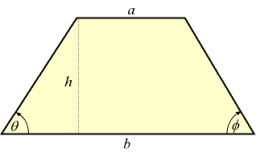# Isosceles 7929

ABCD isosceles trapezoid. A = 6cm, e = 7cm and delta angle = 105 °. Calculate the remaining pages.

b =  5.4788 cm
c =  3.164 cm
d =  5.4788 cm

### Step-by-step explanation:

Our quadratic equation calculator calculates it.

Try calculation via our triangle calculator.Did you find an error or inaccuracy? Feel free to write us. Thank you!

Tips for related online calculators
Calculation of an isosceles triangle.
Cosine rule uses trigonometric SAS triangle calculator.